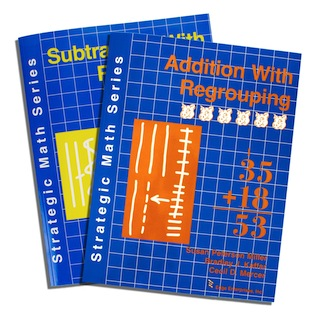Are your students having trouble with addition and subtraction problems that require regrouping? Perhaps they have trouble remembering the sequential steps that need to be followed when solving these types of problems, or maybe they have difficulty deciding when to regroup and when not to regroup. Perhaps some of your students simply subtract the smaller number from the larger number even when the smaller number is in the top number of the problem. These types of errors are very common, but are easily remediated with two programs in the Strategic Math Series: Level 2. These programs are Addition With Regrouping and Subtraction With Regrouping.

The lessons in these books are based on the “concrete-representational-abstract” method of instruction. This means that students first learn to understand and solve computation and word problems using base-ten blocks, then move to using drawings (i.e., squares to represent hundreds, vertical lines to represent tens, and horizontal lines to represent ones), and finally work with numbers alone. To help facilitate the transition from using drawings to using only numbers to solve regrouping problems, the students learn a simple strategy.

Each book comes with learning sheets for students to use to practice specific types of regrouping computation and word problems, a pair of cheerful “pig dice” for playing math games in later lessons, and special pages that enable them to practice discriminating problems that require regrouping from those that do not and increase their speed at coming up with the correct answers. Save money by ordering both manuals at the same time. These books can be used either as part of a basic math curriculum for grades 1-6, or as a remedial program for older students who still need help with regrouping skills.

• Sort by Default
• Display 15 Products per page